## 9.2.2 Nondeterministic vs. Probabilistic Models

What is the best decision for the robot, given that it is engaged in a game against nature? This depends on what information the robot has regarding how nature chooses its actions. It will always be assumed that the robot does not know the precise nature action to be chosen; otherwise, it is pointless to define nature. Two alternative models that the robot can use for nature will be considered. From the robot's perspective, the possible models are

1. [] Nondeterministic: I have no idea what nature will do.
2. [] Probabilistic: I have been observing nature and gathering statistics.
Under both models, it is assumed that the robot knows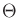in Formulation 9.3 or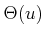for all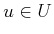in Formulation 9.4. The nondeterministic and probabilistic terminology are borrowed from Erdmann . In some literature, the term possibilistic is used instead of nondeterministic. This is an excellent term, but it is unfortunately too similar to probabilistic in English.

Assume first that Formulation 9.3 is used and that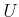andare finite. Under the nondeterministic model, there is no additional information. One reasonable approach in this case is to make a decision by assuming the worst. It can even be imagined that nature knows what action the robot will take, and it will spitefully choose a nature action that drives the cost as high as possible. This pessimistic view is sometimes humorously referred to as Murphy's Law (If anything can go wrong, it will.'')  or Sod's Law. In this case, the best action,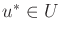, is selected as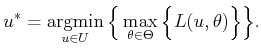(9.14)

The action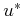is the lowest cost choice using worst-case analysis. This is sometimes referred to as a minimax solution because of the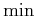and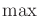in (9.14). Iforis infinite, then theormay not exist and should be replaced by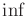or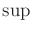, respectively.

Worst-case analysis may seem too pessimistic in some applications. Perhaps the assumption that all actions inare equally likely may be preferable. This can be handled as a special case of the probabilistic model, which is described next.

Under the probabilistic model, it is assumed that the robot has gathered enough data to reliably estimate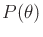(or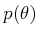ifis continuous). In this case, it is imagined that nature applies a randomized strategy, as defined in Section 9.1.3. It assumed that the applied nature actions have been observed over many trials, and in the future they will continue to be chosen in the same manner, as predicted by the distribution. Instead of worst-case analysis, expected-case analysis is used. This optimizes the average cost to be received over numerous independent trials. In this case, the best action,, is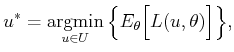(9.15)

in which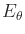indicates that the expectation is taken according to the probability distribution (or density) over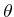. Sinceandtogether form a probability space,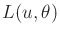can be considered as a random variable for each value of(it assigns a real value to each element of the sample space).9.3 Using, the expectation in (9.15) can be expressed as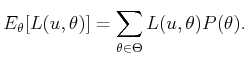(9.16)

Example 9..9 (Nondeterministic vs. Probabilistic)   Return to Example 9.8. Let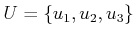represent the robot actions, and let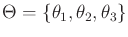represent the nature actions.

Under the nondeterministic model of nature,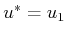, which results in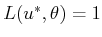in the worst case using (9.14). Under the probabilistic model, let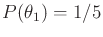,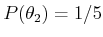, and. To find the optimal action, (9.15) can be used. This involves computing the expected cost for each action: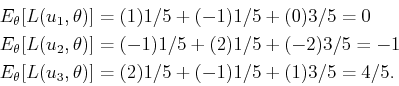(9.17)

The best action is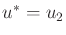, which produces the lowest expected cost,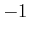.

If the probability distribution had instead been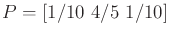, thenwould have been obtained. Hence the best decision depends on; if this information is statistically valid, then it enables more informed decisions to be made. If such information is not available, then the nondeterministic model may be more suitable.

It is possible, however, to assignas a uniform distribution in the absence of data. This means that all nature actions are equally likely; however, conclusions based on this are dangerous; see Section 9.5.In Formulation 9.4, the nature action spacedepends on, the robot action. Under the nondeterministic model, (9.14) simply becomes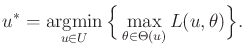(9.18)

Unfortunately, these problems do not have a nice matrix representation because the size ofcan vary for different. In the probabilistic case,is replaced by a conditional probability distribution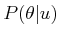. Estimating this distribution requires observing numerous independent trials for each possible. The behavior of nature can now depend on the robot action; however, nature is still characterized by a randomized strategy. It does not adapt its strategy across multiple trials. The expectation in (9.16) now becomes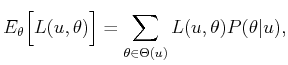(9.19)

which replacesby.

Subsections
Steven M LaValle 2020-08-14